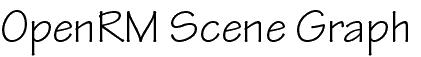## Index of OpenRM - RMAUX Library

```
void rmauxArcBall (float *x1,
float *y1,
float *x2,
float *y2,
RMmatrix *result)
```
``` float *x1, *y1 - pointers to floating point values in the range
(-1..1).  These values define a pixel coordinate position within
the viewport (NDC).  This point is the starting point for when
computing a rotation. (input)
float *x2, *y2 - pointers to floating point values in the range
(-1..1).  These values define a pixel coordinate position within
the viewport (NDC).  This point is the ending point for computing
a rotation. (input)
RMmatrix *result - pointer to an RMmatrix (result). The rotation matrix
computed by rmauxArcBall is copied into this return parameter.
```

The virtual arcball interface implements a rotation model such that changes about the horizontal axis map to X-axis rotations (roughly) and changes about the vertical axis map (roughly) to Y-axis rotations.

The points (x1,y1) and (x2,y2) are projected onto the surface of a sphere, then a quaternion (the result of this routine) is computed that reflects a rotation from point (x1,y1) to point (x2,y2) along a great-arc path on the surface of a sphere.

The rotation matrix computed from (x1,y1) and (x2,y2) is copied into the RMmatrix parameter "return".

librmaux library source file: rmarcball.c
```
void
rmauxDolly (RMcamera3D *toModify,
float *x1,
float *y1,
float *x2,
float *y2)
```
``` RMcamera3D *toModify - a handle to an RMcamera3D. the eye point of
the RMcamera3D is modified by this routine (modified).
float *x1, *y1 - pointers to floating point values in the range
(-1..1).  These values define a pixel coordinate position within
the viewport (NDC).  This point is the starting point for
computing the dolly translation. (input)
float *x2, *y2 - pointers to floating point values in the range
(-1..1).  These values define a pixel coordinate position within
the viewport (NDC).  This point is the ending point for computing
the dolly translation. (input)
```

The virtual dolly interface implements a camera dollying motion such that changes in the vertical directions exponentially control the camera dolly translation. Positive motions up dolly the camera towards the look-at and negative motions down dolly the camera away from the camera look-at.

This routine modifies the eye point of the toModify RMcamera3D parameter.

librmaux library source file: rmarcball.c
```
void
rmauxTranslate (RMcamera3D *toModify,
float *x1,
float *y1,
float *x2,
float *y2)
```
``` RMcamera3D *toModify - a handle to an RMcamera3D object (modified).
The eye and look-at points of this parameter are modified by
this routine.
float *x1, *y1 - pointers to floating point values in the range
(-1..1).  These values define a pixel coordinate position within
the viewport (NDC).  This point is the starting point for
computing the image plane translation. (input)
float *x2, *y2 - pointers to floating point values in the range
(-1..1).  These values define a pixel coordinate position within
the viewport (NDC).  This point is the ending point for computing
the image plane translation. (input)
```

The virtual image plane translation implements a translation motion in the image plane of the camera. Motions in the window correspond directly to the image plane translation applied to the camera eye and look-at points.

This routine will modify the eye and look-at points of the input RMcamera3D object.

librmaux library source file: rmarcball.c